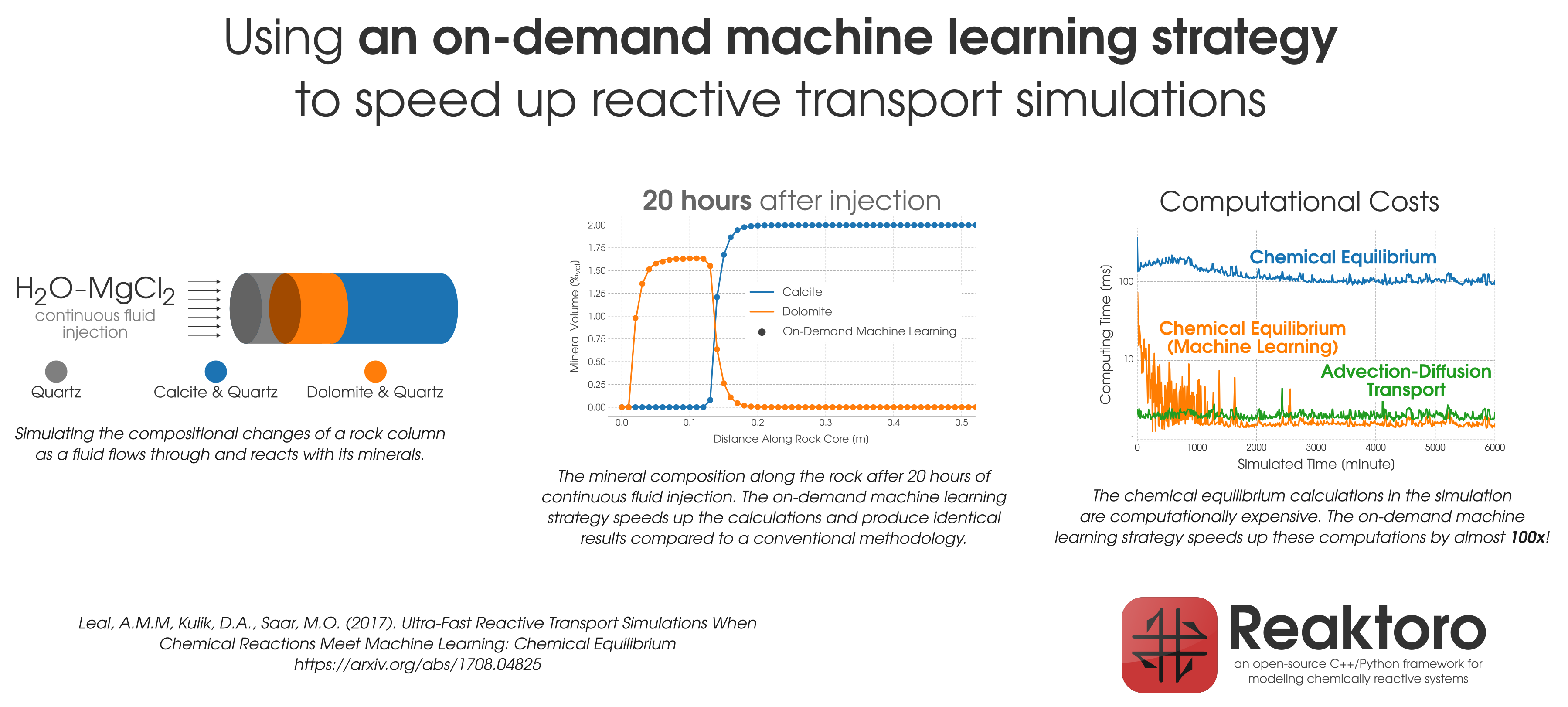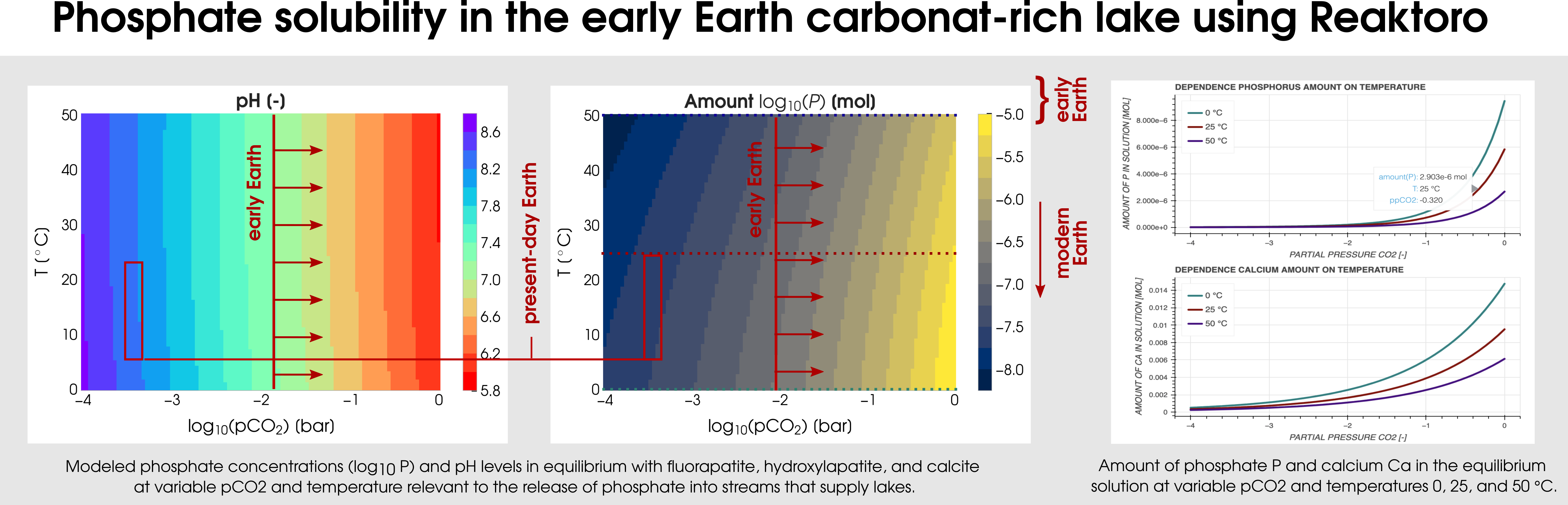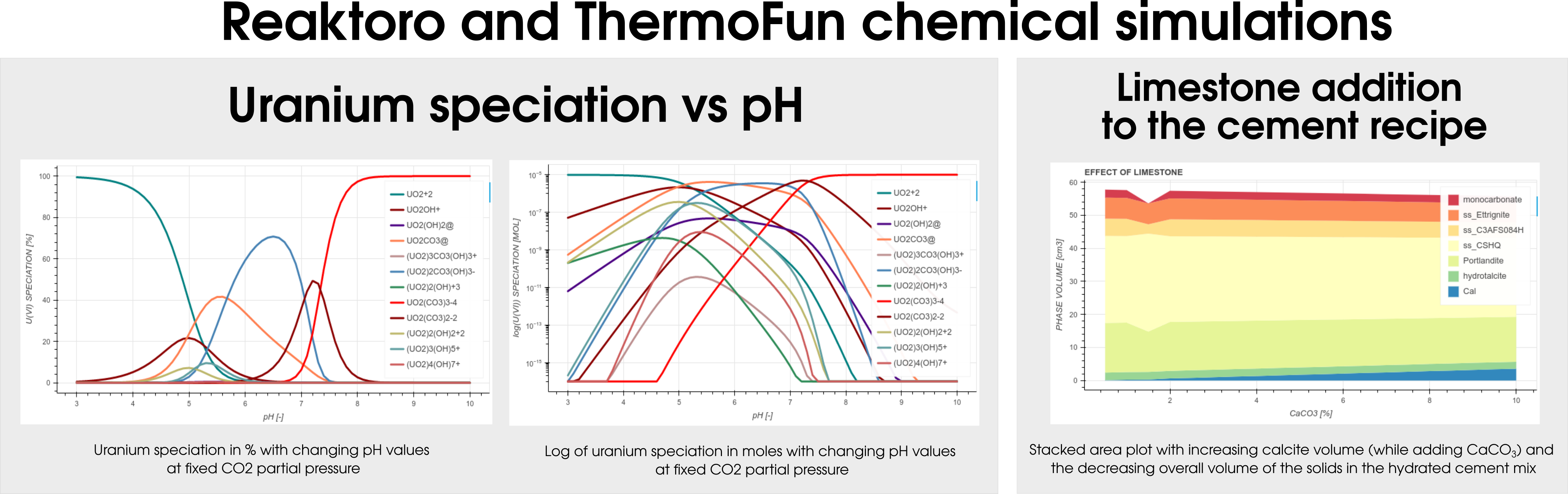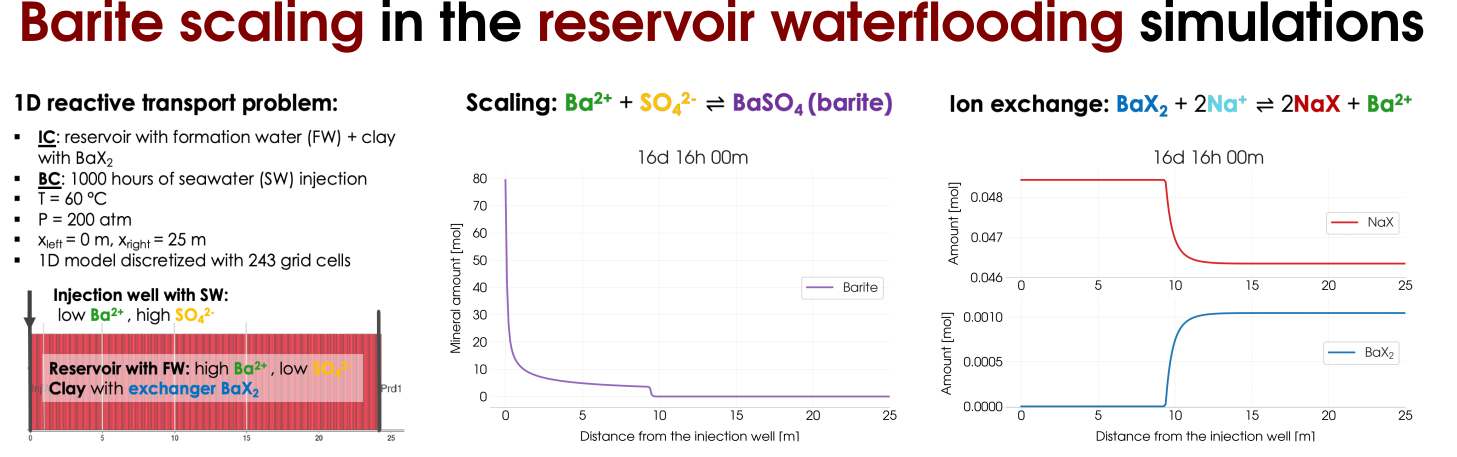# PROJECTS

### Software Development: Reaktoro, a unified framework for modeling chemically reactive systems

Principal Investigator
Dr. Allan Leal, ETH Zurich
Co-Principal Investigator
Researchers
Dr. Svetlana Kyas, ETH Zurich
Start date
September 2015
Current funding
• Swiss National Science Foundation
• Werner Siemens Foundation
• Shell
Supervisors
Dr. Allan Leal, ETH Zurich
Prof. Martin O. Saar, ETH Zurich

#### Description

Reaktoro is a computational framework developed in C++ and Python that implements numerical methods for modeling chemically reactive processes governed by either chemical equilibria, chemical kinetics, or both.

The chemical simulator of Reaktoro is based on the Gibbs energy minimization (GEM) methods as well as a revised law of mass action (rLMA) approach that combines the advantages of both GEM and LMA methods. Recently, on-demand machine learning (ODML) of fast and efficient chemical equilibrium calculations was introduced in Reaktoro.

Chemical equilibrium calculations are essential for many chemical reaction modeling problems. Reaktoro’s computational chemical equilibrium capabilities using Gibbs energy minimization algorithms can be applied to solve a variety of modeling problems. However, sometimes the chemical equilibrium model is not sufficient to understand a chemically reactive process. This happens when we need to understand how the composition of the chemical system changes with time as a result of chemical reactions. For this, chemical kinetics is imperative. Reaktoro can perform chemical kinetics calculations combined with chemical equilibrium (i.e., part of the chemical system evolves under kinetics, while the other is continuously in equilibrium at all times). This mode of calculation is particularly useful for simulating chemically reactive systems in which some reactions have rates that are many orders of magnitude higher than others (and thus can be assumed in instantaneous equilibrium at any time). Finally, chemical equilibrium and kinetics calculations are both space independent. If you need to model transport processes (e.g., advection, diffusion) combined with chemical reactive processes, then chemical transport (or reactive transport) simulations are what you need.

Various interactive examples and tutorials can be found by clicking the button below.#### On-Demand Machine Learning (ODML) approachFigure 1: Acceleration of the chemical equilibration calculation in reactive transport modeling of the dolomitization process.

During reactive transport modeling, the computing cost associated with chemical equilibrium calculations can be 10 to 10,000 times higher than that of fluid flow, heat transfer, and species transport computations. These calculations are performed at least once per mesh cell and once per time step, amounting to billions of them throughout the simulation employing high-resolution meshes. To radically reduce the computing cost of chemical equilibrium calculations (each requiring an iterative solution of a system of non-linear equations), an on-demand machine learning (ODML) algorithm has been introduced in [Leal etal 2020]. The method enables quick and accurate prediction of new chemical equilibrium states using the results of previously solved chemical equilibrium problems within the same reactive transport simulation. The training operations occur on-demand, rather than before the start of the simulation when it is not clear how many training points are needed to accurately and reliably predict all possible chemical conditions that may occur during the simulation. Each on-demand training operation consists of fully solving the equilibrium problem and storing some key information about the just computed chemical equilibrium state (which is used subsequently to rapidly predict similar states whenever possible). We study the performance of the on-demand learning algorithm, which is mass conservative by construction, by applying it to a reactive transport modeling example and achieve a speed-up of one or two orders of magnitude (depending on the activity model used). The implementation and numerical tests are carried out in Reaktoro, a unified open-source framework for modeling chemically reactive systems.

#### The ODML approach in application to the heterogeneous  problems

The ODML  has been thoroughly tested on the reactive transport problems in heterogeneous porous media. The numerical tests are carried out using the coupling of Reaktoro to the open-source software package Firedrake. The numerical results obtained demonstrate how relatively complex simulations of fluid flow coupled with reactive transport can be performed substantially faster by means of radically decreasing the computing costs of chemical reaction calculations (see Figure 2).(a)(b)

Figure 2: Simulation of (a) the dolomitization process and (b) the hydrogen sulfide scavenging in the heterogeneous media together with the summary of the ODML method’s performance.

Extentions of the  ODML approach for chemical kinetics

Besides, we are working on an extension of the ODML approach to support kinetically controlled reactions. We also consider further investigations with more complex geochemical and geological conditions. We plan to extend Reaktoro’s functionality to model reservoirs souring as a result of the activities of sulfide-reducing bacteria, mixing of the groundwater and seawater in the oil reservoir as well as scaling effects this process results to, modeling the effects that seawater or sodium chloride have during the cement rock attack, among many more. An extension to the three-dimensional problem with heterogeneity will require the further implementation of a stable numerical scheme to solve the Darcy problem. To enable full coupling of the transport and flow problems, we plan to continue developing the reactive transport simulator and present obtained results in the future articles.

Reaktoro v2.0 for geobiological simulations

Reaktoro v2.0 can be used for various geobiological simulations. One of them is the modelingof carbonate-rich lakes, which were relatively common on the early Earth. The Earth’s CO2-rich atmosphere can be modeled by fixing the fugacity of the simulated chemical states. In particular, a consequence of early Earth’sCO2-rich atmosphere (corresponding to the partial pressure of CO2 from -2 to 0) is that it would have enhanced the weathering of hydroxyl- and fluorapatite in mafic rocks by lowering the pH of surface waters. Figure 3 plots the two-dimensional dependence of the phosphate solubility and pH on the range of partial CO2 pressures and temperatures.Figure 3: Calculation of the phosphate solubility in the early Earth carbonat-rich lake using Reaktoro

Reaktoro v2.0 usage for different applications using the ThermoFun

Among many databases, Reaktoro v2.0 allows modeling geochemical applications using ThermoFun, a general-purpose open-source client that provides thermodynamic properties of substancesand reactions at the desired temperature and pressure. Figure 4 below demonstrates the result of the calculation of uranium speciation with changing pH values at fixed CO2partial pressure (left-hand side) and simulations of the limestone addition to the cement formulation (right-hand side).Figure 4: Illustraton of the different colloborations projects between Reaktoro and Thermofun.

Barite scalling including the ion-exchange effects on therock surface

Barite scaling can be considered as a side effect that occurs during waterflooding of the oil reser-voirs the result of the contrasting compositions of the injected seawater (SW) fluid and the forma-tion water (FW) in the reservoir that coexists with oil. The precipitation of barite BaSO4 happens according to the reaction combining  Ba2+ions are present in the FW and abundant SO2−4 ions from the injected seawater. In addition, we assume the presence of clay (an ion-exchange site X− occupied by Na+ions ) in the reservoir. Therefore, in addition to the mineral scaling the ion-exchange reaction. Schematically, we illustrate the reactive transport experiment in Figure 5 (left) and the results of the scaling as well as the ion-exchange reactions are presented on the right-hand side of  Figure 5.Figure 5: Reactive transort modelling of the barite scaling in the reservoir waterflooding including the ion-exchange processes.

#### Acknowledgments

Reaktoro would not exist without its past and current supporters and contributors, who supported the project with either financial, scientific, and/or coding input.

• Imperial College London, UK (2011-2014), Qatar Carbonates and Carbon Storage Research Centre (QCCSRC)
• Paul Scherrer Institute, Switzerland (2014-2015), Laboratory for Waste Management

Industries

Reaktoro is proud of its past and current financial support from industries. We would like to thank:

• Shell (2011-2014, 2019-present), Shell Technology Centre Amsterdam
• Qatar Petroleum and Qatar Science & Technology Park (2011-2014), Qatar Carbonates and Carbon Storage Research Centre (QCCSRC)

Related Publications by the GEG Group

### REFEREED PUBLICATIONS IN JOURNALS

Leal, A.M.M., S. Kyas, D. Kulik, and M.O. Saar, Accelerating Reactive Transport Modeling: On‑Demand Machine Learning Algorithm for Chemical Equilibrium Calculations, Transport in Porous Media, 133, pp. 161-204, 2020. [View Abstract]During reactive transport modeling, the computing cost associated with chemical equilibrium calculations can be 10 to 10,000 times higher than that of fluid flow, heat transfer, and species transport computations. These calculations are performed at least once per mesh cell and once per time step, amounting to billions of them throughout the simulation employing high-resolution meshes. To radically reduce the computing cost of chemical equilibrium calculations (each requiring an iterative solution of a system of nonlinear equa-tions), we consider an on-demand machine learning algorithm that enables quick and accu-rate prediction of new chemical equilibrium states using the results of previously solved chemical equilibrium problems within the same reactive transport simulation. The training operations occur on-demand, rather than before the start of the simulation when it is not clear how many training points are needed to accurately and reliably predict all possible chemical conditions that may occur during the simulation. Each on-demand training opera-tion consists of fully solving the equilibrium problem and storing some key information about the just computed chemical equilibrium state (which is used subsequently to rap-idly predict similar states whenever possible). We study the performance of the on-demand learning algorithm, which is mass conservative by construction, by applying it to a reactive transport modeling example and achieve a speed-up of one or two orders of magnitude (depending on the activity model used). The implementation and numerical tests are car-ried out in Reaktoro (reakt oro.org), a unified open-source framework for modeling chemi-cally reactive systems.

Miron, G. D., A.M.M. Leal, and A. Yapparova, Thermodynamic Properties of Aqueous Species Calculated Using the HKF Model: How Do Different Thermodynamic and Electrostatic Models for Solvent Water Affect Calculated Aqueous Properties?, Geofluids, a43, pp. 1-24, 2019. [View Abstract]Thermodynamic properties of aqueous species are essential for modeling of fluid-rock interaction processes. The Helgeson-Kirkham-Flowers (HKF) model is widely used for calculating standard state thermodynamic properties of ions and complexes over a wide range of temperatures and pressures. To do this, the HKF model requires thermodynamic and electrostatic models of water solvent. In this study, we investigate and quantify the impact of choosing different models for calculating water solvent volumetric and dielectric properties, on the properties of aqueous species calculated using the HKF model. We identify temperature and pressure conditions at which the choice of different models can have a considerable effect on the properties of aqueous species and on fluid mineral equilibrium calculations. The investigated temperature and pressure intervals are 25–1000°C and 1–5 kbar, representative of upper to middle crustal levels, and of interest for modeling ore-forming processes. The thermodynamic and electrostatic models for water solvent considered are: Haar, Gallagher and Kell (1984), Wagner and Pruß (2002), and Zhang and Duan (2005), to calculate water volumetric properties, and Johnson and Norton (1991), Fernandez and others (1997), and Sverjensky and others (2014), to calculate water dielectric properties. We observe only small discrepancies in the calculated standard partial molal properties of aqueous species resulting from using different water thermodynamic models. However, large differences in the properties of charged species can be observed at higher temperatures (above 500°C) as a result of using different electrostatic models. Depending on the aqueous speciation and the reactions that control the chemical composition, the observed differences can vary. The discrepancy between various electrostatic models is attributed to the scarcity of experimental data at high temperatures. These discrepancies restrict the reliability of the geochemical modeling of hydrothermal and ore formation processes, and the retrieval of thermodynamic parameters from experimental data at elevated temperatures and pressures.

Walsh, S.D.C., N. Garapati, A.M.M. Leal, and M.O. Saar, Calculating thermophysical fluid properties during geothermal energy production with NESS and Reaktoro, Geothermics, 70, pp. 146-154, 2017. [View Abstract]We investigate how subsurface fluids of different compositions affect the electricity generation of geothermal power plants. First, we outline a numerical model capable of accounting for the thermophysical properties of geothermal fluids of arbitrary composition within simulations of geothermal power production. The behavior of brines with varying compositions from geothermal sites around the globe are then examined using the model. The effect of each brine on an idealized binary geothermal power plant is simulated, and their performances compared by calculating the amount of heat exchanged from the fluid to the plant's secondary cycle. Our simulations combine (1) a newly developed Non-linear Equation System Solver (NESS), for simulating individual geothermal power plant components, (2) the advanced geochemical speciation solver, Reaktoro, used for calculation of thermodynamic fluid properties, and (3) compositional models for the calculation of fluid-dynamical properties (e.g., viscosity as a function of temperature and brine composition). The accuracy of the model is verified by comparing its predictions with experimental data from single-salt, binary-salt, and multiple-salt solutions. The geothermal power plant simulations show that the brines considered in this study can be divided into three main categories: (1) those of largely meteoric origin with low salinity for which the effect of salt concentration is negligible; (2) moderate-depth brines with high concentrations of Na+ and K+ ions, whose performance is well approximated by pure NaCl solutions of equivalent salinity; and (3) deeper, high-salinity brines that require a more detailed consideration of their composition for accurate simulation of plant operations.

Leal, A.M.M., D.A. Kulik, W.R. Smith, and M.O. Saar, An overview of computational methods for chemical equilibrium and kinetic calculations for geochemical and reactive transport modeling, Pure and Applied Chemistry, 89/5, pp. 597-643, 2017. [View Abstract]We present an overview of novel numerical methods for chemical equilibrium and kinetic calculations for complex non-ideal multiphase systems. The methods we present for equilibrium calculations are based either on Gibbs energy minimization (GEM) calculations or on solving the system of extended law of mass-action (xLMA) equations. In both methods, no a posteriori phase stability tests, and thus no tentative addition or removal of phases during or at the end of the calculations, are necessary. All potentially stable phases are considered from the beginning of the calculation, and stability indices are immediately available at the end of the computation to determine which phases are actually stable at equilibrium. Both GEM and xLMA equilibrium methods are tailored for computationally demanding applications that require many rapid local equilibrium calculations, such as reactive transport modeling. The numerical method for chemical kinetic calculations we present supports both closed and open systems, and it considers a partial equilibrium simplification for fast reactions. The method employs an implicit integration scheme that improves stability and speed when solving the often stiff differential equations in kinetic calculations. As such, it requires compositional derivatives of the reaction rates to assemble the Jacobian matrix of the resultant implicit algebraic equations that are solved at every time step. We present a detailed procedure to calculate these derivatives, and we show how the partial equilibrium assumption affects their computation. These numerical methods have been implemented in Reaktoro (reaktoro.org), an open-source software for modeling chemically reactive systems. We finish with a discussion on the comparison of these methods with others in the literature.

Leal, A.M.M., D.A. Kulik, and G. Koskowski, Computational methods for reactive transport modeling: A Gibbs energy minimization approach for multiphase equilibrium calculations, Advances in Water Resources, 88, pp. 231-240, 2016. [View Abstract]We present a numerical method for multiphase chemical equilibrium calculations based on a Gibbs energy minimization approach. The method can accurately and efficiently determine the stable phase assemblage at equilibrium independently of the type of phases and species that constitute the chemical system. We have successfully applied our chemical equilibrium algorithm in reactive transport simulations to demonstrate its effective use in computationally intensive applications. We used FEniCS to solve the governing partial differential equations of mass transport in porous media using finite element methods in unstructured meshes. Our equilibrium calculations were benchmarked with GEMS3K, the numerical kernel of the geochemical package GEMS. This allowed us to compare our results with a well-established Gibbs energy minimization algorithm, as well as their performance on every mesh node, at every time step of the transport simulation. The benchmark shows that our novel chemical equilibrium algorithm is accurate, robust, and efficient for reactive transport applications, and it is an improvement over the Gibbs energy minimization algorithm used in GEMS3K. The proposed chemical equilibrium method has been implemented in Reaktoro, a unified framework for modeling chemically reactive systems, which is now used as an alternative numerical kernel of GEMS.

Leal, A.M.M., D.A. Kulik, and M.O. Saar, Enabling Gibbs energy minimization algorithms to use equilibrium constants of reactions in multiphase equilibrium calculations, Chemical Geology, 437, pp. 170-181, 2016. [View Abstract]The geochemical literature provides numerous thermodynamic databases compiled from equilibrium constants of reactions. These databases are typically used in speciation calculations based on the law of mass action (LMA) approach. Unfortunately, such LMA databases cannot be directly used in equilibrium speciation methods based on the Gibbs energy minimization (GEM) approach because of their lack of standard chemical potentials of species. Therefore, we present in this work a simple conversion approach that calculates apparent standard chemical potentials of species from equilibrium constants of reactions. We assess the consistency and accuracy of the use of apparent standard chemical potentials in GEM algorithms by benchmarking equilibrium speciation calculations using GEM and LMA methods with the same LMA database. In all cases, we use PHREEQC to perform the LMA calculations, and we use its LMA databases to calculate the equilibrium constants of reactions. GEM calculations are performed using a Gibbs energy minimization method of Reaktoro — a unified open-source framework for numerical modeling of chemically reactive systems. By comparing the GEM and LMA results, we show that the use of apparent standard chemical potentials in GEM methods produces consistent and accurate equilibrium speciation results, thus validating our new, practical conversion technique that enables GEM algorithms to take advantage of many existing LMA databases, consequently extending and diversifying their range of applicability.

Leal, A.M.M., D. Kulik, G. Kosakowski, and M.O. Saar, Computational methods for reactive transport modeling: An extended law of mass-action, xLMA, method for multiphase equilibrium calculations, Advances in Water Resources, 96, pp. 405-422, 2016. [View Abstract]We present a numerical method for multiphase chemical equilibrium calculations based on a Gibbs energy minimization approach. The method can accurately and efficiently determine the stable phase assemblage at equilibrium independently of the type of phases and species that constitute the chemical system. We have successfully applied our chemical equilibrium algorithm in reactive transport simulations to demonstrate its effective use in computationally intensive applications. We used FEniCS to solve the governing partial differential equations of mass transport in porous media using finite element methods in unstructured meshes. Our equilibrium calculations were benchmarked with GEMS3K, the numerical kernel of the geochemical package GEMS. This allowed us to compare our results with a well-established Gibbs energy minimization algorithm, as well as their performance on every mesh node, at every time step of the transport simulation. The benchmark shows that our novel chemical equilibrium algorithm is accurate, robust, and efficient for reactive transport applications, and it is an improvement over the Gibbs energy minimization algorithm used in GEMS3K. The proposed chemical equilibrium method has been implemented in Reaktoro, a unified framework for modeling chemically reactive systems, which is now used as an alternative numerical kernel of GEMS.

Leal, A.M.M., M.J. Blunt, and T.C. LaForce, A chemical kinetics algorithm for geochemical modelling, Applied Geochemistry, 55, pp. 46-61, 2015. [View Abstract]A chemical kinetics algorithm is presented for geochemical applications. The algorithm is capable of handling both equilibrium- and kinetically-controlled reactions in multiphase systems. The ordinary differential equations (ODEs) are solved using an implicit multistep backward differentiation formula (BDF) algorithm to ensure efficiency and stability when integrating stiff ODEs. An adaptive control scheme of the time step is adopted to guarantee small steps in steeper regions and large steps in smoother regions of the integration. Analytical derivatives of the reaction rates and species activities are used to permit the use of larger time steps, and to increase the robustness of the calculations. The chemical equilibrium calculations are performed using a Gibbs free energy minimisation algorithm, which is based on a trust-region interior-point method adapted with a watchdog strategy that yields quadratic rates of convergence near the solution. The chemical kinetics algorithm is applied to geochemical problems relevant to carbon storage in saline aquifers. The calculations assume aqueous, gaseous and mineral phases, where the kinetics of the waterâ€“gasâ€“rock interactions are investigated. The results allow us to estimate the time frames at which brine of different salinities and supercritical CO2 attains equilibrium with a carbonate rock, as well as the amount of carbon dioxide trapped by solubility and mineralisation mechanisms.

#### References

eal, A. M. M., Kyas, S., Kulik, D. A., & Saar, M. O. (2020). Accelerating Reactive Transport Modeling: On-Demand Machine Learning Algorithm for Chemical Equilibrium Calculations. Transport in Porous Media, 133(2), 161–204. https://doi.org/10.1007/s11242-020-01412-1

Leal, A. M. M., Kyas, S., Kulik, D. A., & Saar, M. O. (2020). Accelerating Reactive Transport Modeling: On-Demand Machine Learning Algorithm for Chemical Equilibrium Calculations. Transport in Porous Media, 133(2), 161–204. https://doi.org/10.1007/s11242-020-01412-1

Leal, A. M. M., Kulik, D. A., Smith, W. R., & Saar, M. O. (2017). An overview of computational methods for chemical equilibrium and kinetic calculations for geochemical and reactive transport modeling. Pure and Applied Chemistry, 89(5), 597–643. https://doi.org/10.1515/pac-2016-1107

Leal, A. M. (2015). Reaktoro: A unified framework for modeling chemically reactive systems, reaktoro.org.